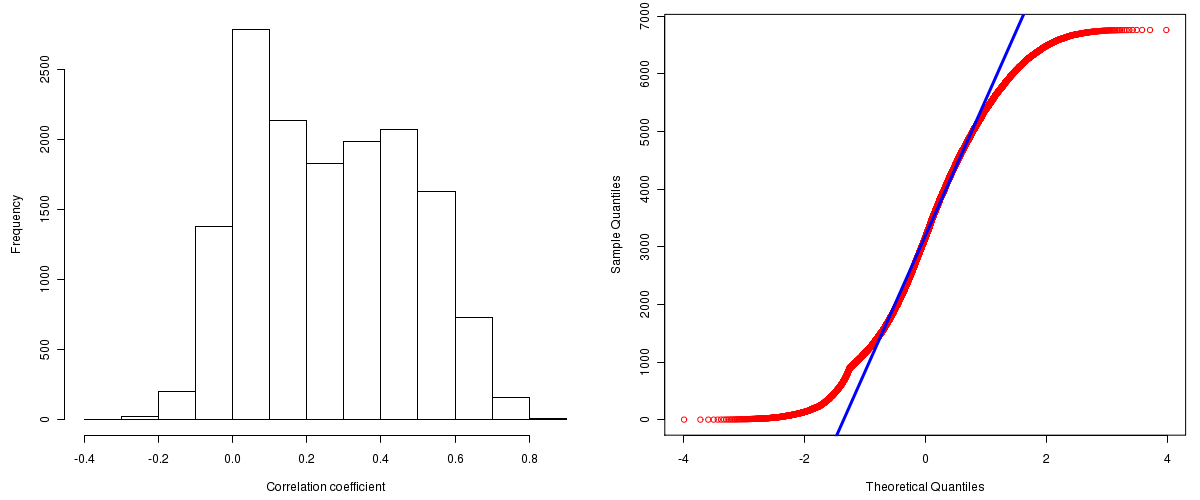PANCANCER: Correlations between copy number and mRNA expression
Maintained by John Zhang (Dana-Farber Cancer Institute)
Overview
Introduction

A TCGA sample is profiled to detect the copy number variations and expressions of genes. This pipeline attempts to correlate copy number and expression data of genes across samples to determine if the copy number variations also result in differential expressions. This report contains the calculated correlation coefficients based on measurements of genomic copy number (log2) values and intensity of the expressions of the corresponding feature across patients. High positive/low negative correlation coefficients indicate that genomic alterations result in differences in the expressions of mRNA the genomic regions transcribe.

Summary

The correlation coefficients in 10, 20, 30, 40, 50, 60, 70, 80, 90 percentiles are -0.00404, 0.04782, 0.104, 0.17178, 0.2493, 0.3319, 0.40534, 0.47608, 0.55744, respectively.

Results
Correlation results

Number of genes and samples used for the calculation are shown in Table 1. Figure 1 shows the distribution of calculated correlation coefficients and quantile-quantile plot of the calculated correlation coefficients against a normal distribution. Table 2 shows the top 20 features ordered by the value of correlation coefficients.

Table 1.  Counts of mRNA and number of samples in copy number and expression data sets and common to both

Category Copy number Expression Common
Sample 4422 1645 1500
Genes 29390 17815 15551

Figure 1.  Summary figures. Left: histogram showing the distribution of the calculated correlations across samples for all Genes. Right: QQ plot of the calculated correlations across samples. The QQ plot is used to plot the quantiles of the calculated correlation coefficients against that derived from a normal distribution. Points deviating from the blue line indicate deviation from normality.Table 2.  Get Full Table Top 20 features (defined by the feature column) ranked by correlation coefficients

feature r p-value q-value chrom start end geneid
LSM1 0.8567 0 0 8 38140014 38153183 27257
CLNS1A 0.8302 0 0 11 77004847 77026495 1207
BRF2 0.8176 0 0 8 37820558 37826569 55290
WHSC1L1 0.8175 0 0 8 38251717 38358947 54904
ASH2L 0.8147 0 0 8 38082223 38116216 9070
RPS6KB1 0.8106 0 0 17 55325225 55382569 6198
STARD3 0.8047 0 0 17 35046938 35073263 10948
SNF8 0.8041 0 0 17 44362457 44377153 11267
SENP2 0.8034 0 0 3 186786725 186831583 59343
WDR53 0.7943 0 0 3 197765456 197779810 348793
DDX47 0.7932 0 0 12 12857547 12874182 51202
PPFIA1 0.7914 0 0 11 69794471 69908150 8500
C17orf37 0.7874 0 0 17 35138935 35140314 84299
CNOT2 0.7836 0 0 12 68923489 69033985 4848
C19orf12 0.7795 0 0 19 34883910 34897971 83636
SAP18 0.7777 0 0 13 20612685 20620977 10284
PSMD8 0.7775 0 0 19 43557061 43566304 5714
GTF2F2 0.7772 0 0 13 44592672 44756237 2963
SLC35B1 0.7772 0 0 17 45133689 45140281 10237
GSTT1 0.7763 0 0 22 22706141 22714231 2952
Methods & Data
Input

Gene level (TCGA Level III) expression data and copy number data of the corresponding loci derived by using the CNTools package of Bioconductor were used for the calculations. Pearson correlation coefficients were calculated for each pair of genes shared by the two data sets across all the samples that were common.

Correlation across sample

Pairwise correlations between the log2 copy numbers and expressions of each gene across samples were calculated using Pearson correlation.